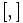# SmallGroup(64,210)

View a complete list of particular groups (this is a very huge list!)[SHOW MORE]

## Definition

This group is defined by the following presentation:$G := \langle a_1,a_2,a_3,a_4 \mid a_1^2 = a_2^2 = a_3^4 = a_4^4 = e, [a_1,a_2] = a_3^2, [a_1,a_3] = e, [a_2,a_3] = a_4^2, [a_1,a_4] = [a_2,a_4] = [a_3,a_4] = e \rangle$

Here$e$ stands for the identity element and$[ , ]$ stands for the commutator of two elements. It does not matter whether we choose the left or right conventions -- the specific presentations differ, but they define isomorphic groups.

## Arithmetic functions

Want to compare and contrast arithmetic function values with other groups of the same order? Check out groups of order 64#Arithmetic functions

## GAP implementation

### Group ID

This finite group has order 64 and has ID 210 among the groups of order 64 in GAP's SmallGroup library. For context, there are groups of order 64. It can thus be defined using GAP's SmallGroup function as:

SmallGroup(64,210)

For instance, we can use the following assignment in GAP to create the group and name it$G$:

gap> G := SmallGroup(64,210);

Conversely, to check whether a given group$G$ is in fact the group we want, we can use GAP's IdGroup function:

IdGroup(G) = [64,210]

or just do:

IdGroup(G)

to have GAP output the group ID, that we can then compare to what we want.

### Description by presentation

gap> F := FreeGroup(4);
<free group on the generators [ f1, f2, f3, f4 ]>
gap> G := F/[F.1^2,F.2^2,F.3^4,F.4^4,Comm(F.1,F.2)*F.3^(-2),Comm(F.1,F.3),Comm(F.2,F.3)*F.4^(-2),Comm(F.1,F.4),Comm(F.2,F.4),Comm(F.3,F.4)];
<fp group on the generators [ f1, f2, f3, f4 ]>
gap> IdGroup(G);
[ 64, 210 ]